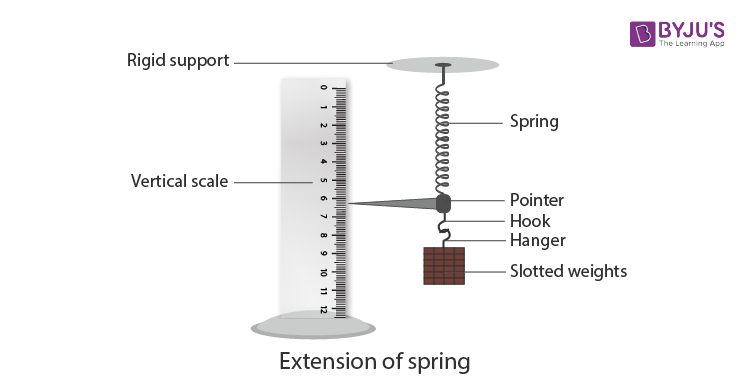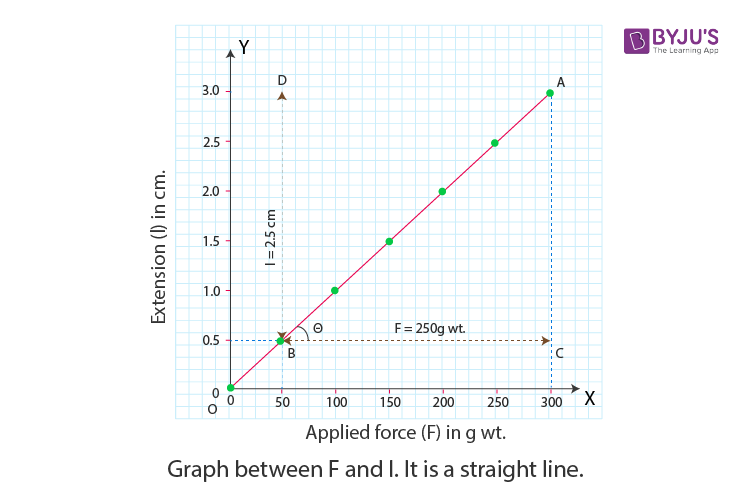# To Find The Force Constant Of A Helical Spring By Plotting A Graph Between Load And Extension

In the class 11 Physics experiment given here, we will be learning how to find the spring constant of a spring by plotting a graph between the load and extension. The Spring constant, also known as the force constant, is the restoring force per unit extension in the spring. Its value is determined by the elastic properties of the spring.

## Aim

To find the force constant of a helical spring by plotting a graph between load and extension.

## Apparatus/ Materials Required

• A rigid support
• Spring
• A 50 g or 20 g hanger
• Six 20 g or 50 g slotted weight
• A fine pointer
• A vertical wooden scale
• A hook

## Diagram## Theory

When a load F suspended from the lower free end of the spring hanging from rigid support increases its length by l, then

$$\begin{array}{l}F\propto l\end{array}$$

or

$$\begin{array}{l}F=Kl\end{array}$$

Where K is the proportionality constant

K, the proportionality constant, is also known as the force constant or the spring constant of the spring

From the above equation, it is clear that if l =1, F = K.

Hence, spring constant can be defined as the force required to produce a unit extension in the spring.

## Procedure

1. Suspend the spring from a rigid support and attach the pointer and the hook from its lower free end.
2. Hang a 50 g hanger from the hook
3. Arrange the vertical wooden scale such that the tip of the pointer comes over the divisions on the scale but does not touch the scale.
5. Gently add a suitable load of 50 g or 20 g of slotted weight to the hanger. The tip of the pointer moves down.
6. Wait for some time till the pointer comes to rest. Repeat step 4.
7. Repeat steps 5 and 6 till six slots have been added.
8. Remove one slotted weight. The pointer moves up. Repeat step 6 and record the reading in the unloading column.
9. Repeat step 8 till the only hanger is left.
10. Record your observation as given below in the table.

## Observations

The least count of vernier scale is 0.1 cm.

 S. No Load on Hanger (W) = Applied Force Reading of Position of the pointer tip Extension (l cm) Loading (x cm) Unloading (y cm) Mean z = (x+y)/2 1 2 3 4 5 6 7 0 50 100 150 200 250 300

## Graph

Plot a graph between F and l with F along the x-axis and l along the y-axis. The graph is a straight line as shown in the figure below.From the graph, it is seen that a change of F from B to C, produces a change of l from B to D. In other words, 250 g of weight produces a 2.5 cm extension. From this given data, the spring constant can be calculated as follows:

$$\begin{array}{l}K=\frac{F}{l}=\frac{BC}{AC}\end{array}$$
$$\begin{array}{l}K=\frac{250}{2.5}=100\,g\,wt\,per\,cm\end{array}$$

## Result

The spring constant of the given spring is 100 g wt per cm.

## Viva Voice

1. What is a rigid body?

Answer: A body is said to be a rigid body when it doesn’t undergo any change in its length, volume or shape on the application of force.

2. What is a deforming force?

Answer: A balanced force that produces deformation in a body is known as the deforming force.

3. Define stress.

Answer: The restoring force exerted per unit area by the molecules of the deformed body is known as stress.

4. Give the unit of stress.

Answer: The unit of stress is newton per metre square (N m–2).

5. Define the yield point.

Answer: The yield point is a stage beyond the elastic limit, up to which there is no more extension but thinning of wire remains uniform.

6. Why do you remove kinks before starting the experiment?